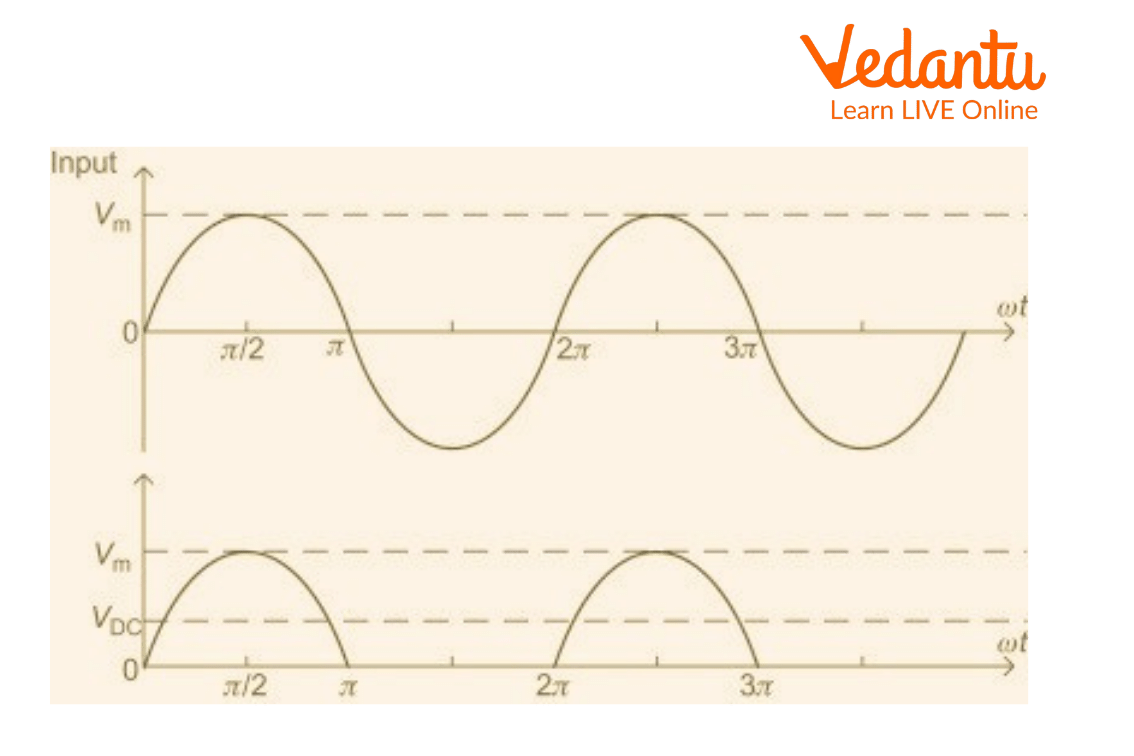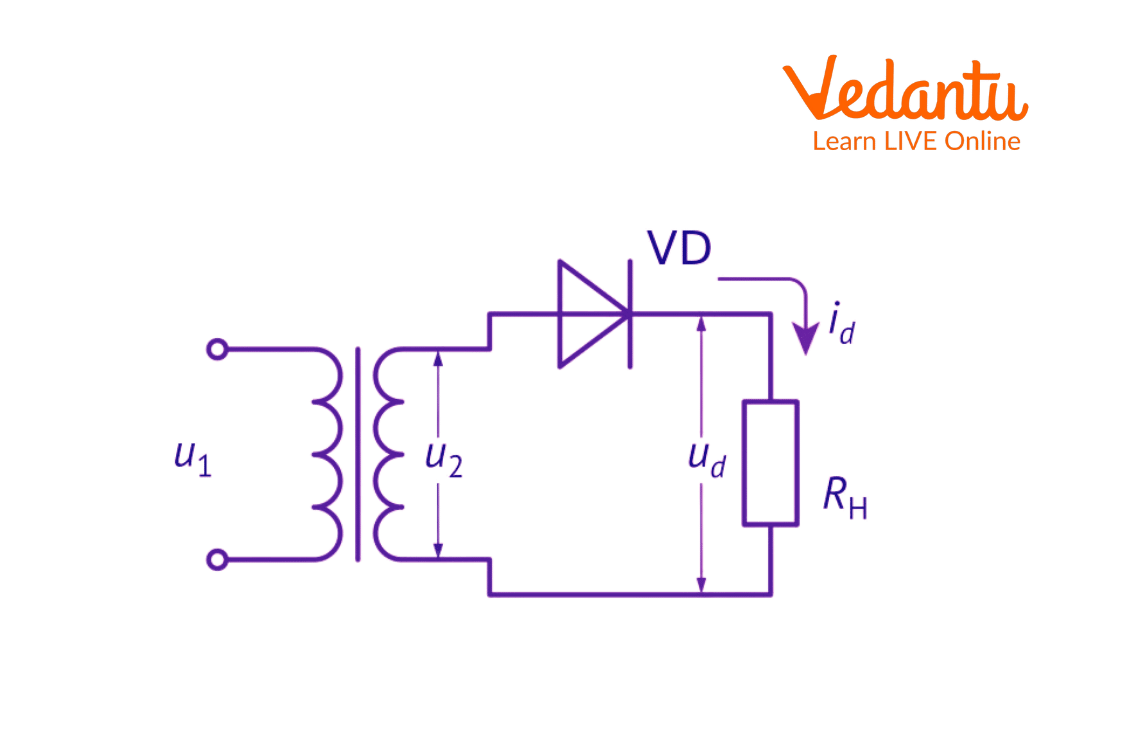Courses
Courses for Kids
Free study material
Free LIVE classes
More

# Half Wave RectifierLIVE
Join Vedantu’s FREE Mastercalss

## What is Half Wave Rectifier? An Introduction

A rectifier uses one or more P-N junction diodes to convert an alternating current (AC) to a direct current (DC). DC voltage is created by half wave rectifiers from AC voltage. In a half wave rectifier circuit, the transformation is carried out by a single diode. An AC voltage waveform can only travel in one half cycle of the input voltage in a half wave rectifier, and blocks the other half of the input voltage.

## Rectifier

• The domestic supply in our nation has a voltage variation of 220 V (RMS) at a frequency of 50 Hz. As DC voltage is needed to run the majority of electronic equipment so the main power supply's AC voltage must be converted to DC voltage hence a rectifier that uses one or more P-N junction diodes is used to change an alternating current (AC) into a direct current (DC).

• The junction diode used in the rectifier has the ability to provide low resistance to the current passing through it when it is forward biased and high resistance to the current passing through it when it is reverse biased.

## Half Wave Rectifier

The most basic type of rectifier is a half wave rectifier, which just needs one diode to be built into a circuit. A single diode coupled in series with a load resistor is exposed to an alternating voltage in a half wave rectifier circuit.Waveform of Half Wave Rectifier

A full wave of an AC signal is made up of two equal positive and negative parts. Only one-half of the input AC cycle is passed by the PN junction diode to conduct electricity. When the diode is forward-based during a positive half cycle, it conducts and transfers the half wave. As the diode has little resistance in forward bias so the load resistor R experiences high current flow. As output voltage V grows across R, the half wave is transferred. When a diode is reverse biased during a negative half cycle, it does not conduct, Therefore, it provides no output (V=0). The circuit is known as a half wave rectifier because the output only has +ve half cycles or unidirectional half waves.

## Half Wave Rectifier Diagram and Parameters

High Ac voltage is given to the primary side of the step down transformer. Secondary low voltage is provided to the diode. During the positive half cycle of the AC voltage, the diode is forward biased, and during the negative half cycle, it is reverse biased.

The waveform of the final output voltage is depicted in the figure below:Half Wave Circuit

There are a few advantages of half wave rectifiers such as simple circuits, low cost, very easy to use, can be constructed easily, requires very few components, and so on. It has a 40.6% maximum efficiency. The half wave rectifier is very helpful in reducing noise.

## Ripple Factor of Half Wave Rectifier

How well a half wave rectifier can change AC voltage into DC voltage is determined by the ripple factor. The formula below can be used to calculate the ripple factor,

$\gamma = \sqrt {{{\left( {\dfrac{{{V_{rms}}}}{{{V_{dc}}}}} \right)}^2}} - 1$

Ripple factor for the Half wave rectifier is 1.21.

## Efficiency of Half Wave Rectifier

The ratio of the DC power output to the AC power input is the measure of a half wave rectifier's efficiency. The following is the half wave rectifier efficiency formula,

$\eta = \dfrac{{{P_{dc}}}}{{{P_{ac}}}}$

The maximum efficiency of the half wave rectifier is 40.6%.

## RMS Value

The half wave rectifier RMS value is given by

RMS = Max. Value/2

VRMS=Vm/2

## Interesting Facts

• In the mosquito repellent, half wave rectifiers are used to form the fumes out of the lead.

• They serve the aim of signal demodulation.

• They are employed in applications for rectification.

• They serve applications involving pulse-generating circuits.

## Solved Problems

1. If 100 watts AC power is applied to the input of the half wave rectifier and obtained dc power is 40 watts, calculate the rectification efficiency.

Sol.

For the half wave rectifier, the rectification efficiency is given by,

${\rm{Rectification efficiency = }}\dfrac{{D{C_{out}}Power}}{{A{C_{in}}Power}}$

$\eta = \dfrac{{{P_{dc}}}}{{{P_{ac}}}}$

$\eta = \dfrac{{40}}{{100}}$

$\eta = 0.4$

% $\eta = 40\%$ (Convert to percentage)

2. If the internal resistance of the diode is 10 W and load resistance is 1000 W for half wave rectifiers, calculate the rectification efficiency. Take Irms=24.75 mA and Idc=15.76 mA.

Sol.

Here, internal resistance = Rd = 10 W

Load resistance = RL = 1000 W

Irms = 24.75 mA

Idc = 15.76 mA

We need to find Pdc and Pac for calculating the rectification efficiency.

Now,

${P_{ac}} = {({I_{rms}})^2}({R_d} + {R_L})$

Putting the values and converting mA to A for Pac,

${P_{ac}} = {(24.75 \times {10^{ - 3}})^2}(10 + 1000)$

${P_{ac}} = 0.618{\rm{ Watts}}$

For Pdc,

${P_{dc}} = {({I_{dc}})^2}({R_L})$

${P_{dc}} = {(15.76 \times {10^{ - 3}})^2}(1000)$

${P_{dc}} = 0.248{\rm{ Watts}}$

We know the rectification efficiency of half wave rectifier is,

$\eta = \dfrac{{{P_{dc}}}}{{{P_{ac}}}}$

$\eta = \dfrac{{0.248}}{{0.618}}$

$\eta = \dfrac{{0.248}}{{0.618}} \times 100{\rm{ (convert to percentage)}}$

$\eta = 40.12\%$

## Summary

Half wave rectifier can be defined as a rectifier that allows only one half-cycle of an AC voltage waveform to pass, and blocks the other half-cycle. Half wave rectifiers are used to convert AC voltages to DC voltages. Half wave rectifiers require only a single diode to construct.

Last updated date: 29th Sep 2023
Total views: 100.2k
Views today: 3.00k

## FAQs on Half Wave Rectifier

1. Give some examples of a rectifier.

There are various rectifiers; some examples include silicon-controlled rectifiers, vacuum tube diodes, solid-state diodes, mercury-arc valves, and so on.

2. Is the half wave rectifier better or the full-wave rectifier?

Full-wave rectifier device converts the AC sine waves into DC whereas a half-wave rectifier device only converts one. The full-wave devices are better for high-current devices whereas half-wave rectifier devices are better for low current applications and also for sharing the same transformer.

3. What is the use of a half wave rectifier?

Half-wave rectifier is used in soldering iron types of circuits and it is also used in mosquito repellent to drive lead for fumes. In electric welding, bridge rectifier circuits are used to supply steady and polarised DC voltage.